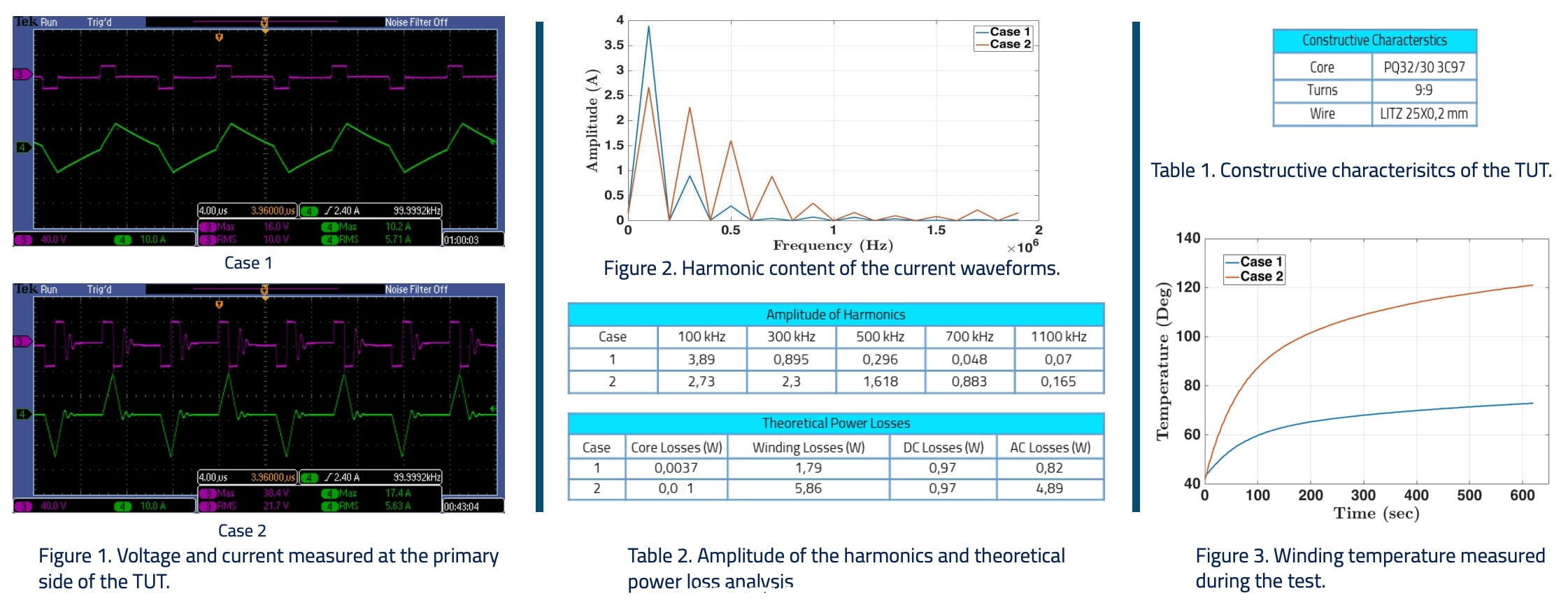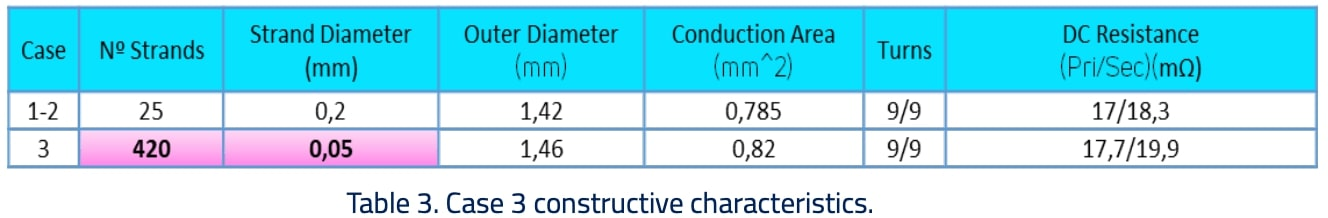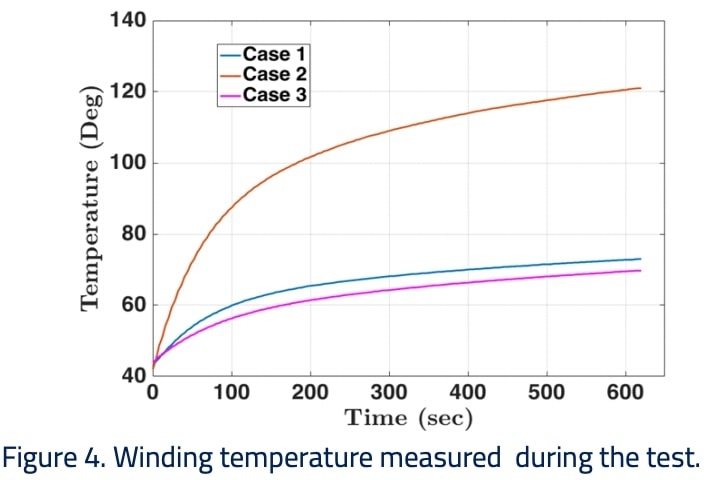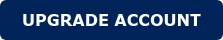Harmonics Impact on the Winding Losses

Real example and harmonics impact at the design stage

The objective of this paper is to show a real example case of how harmonics can considerably affect the performance of a transformer and to provide some guidelines for reducing such impact at the design stage.

Case of study

To evaluate the impact of the harmonics, a transformer was built and tested at Frenetic's Laboratories. Table 1 summarizes the constructive characteristics of the transformer under test (TUT) and Figure 1 shows the waveforms captured with the oscilloscope. Two cases were considered: Case 1 with a clean trapezoidal waveform and Case 2 with a distorted triangular waveform. To have a fair comparison, the same RMS current and therefore same energy content was applied in both cases. It should be noted that although there are some differences in the voltages applied, the core area and number of turns were selected to ensure negligible core losses. Figure 2 and Table 2 show the amplitude of the harmonics measured and the theoretical power losses calculated and Figure 3 shows the temperature measured during the test (thermocouple placed between windings).As it can be seen, having almost the same amplitude at 300 kHz and at the fundamental frequency, and with 50 % of the amplitude at 500 kHz, the theoretical power losses of Case 2 increase more than three times with respect to Case 1. This can be clearly seen in winding temperature measured where 120 ºC are exceeded in less than 10 minutes thus leading to transformer failure.Case study with harmonics considered at the design stage

The same transformer was redesigned optimizing the strand diameter for the 300-700 kHz harmonics. For a fair comparison, the conduction area was kept the same as in cases 1 and 2. The winding losses were reduced down to 1.39W, reaching thus a better performance than both previous cases.Conclusions

Harmonics have a significant impact on magnetic components' performance. This paper has presented a simple example of three cases where the same transformer operates differently under the same input specifications. The objective of this paper is not to think of Litz wire optimization but rather on how the complete system can be optimized by considering the harmonics at the design stage.

You may also like